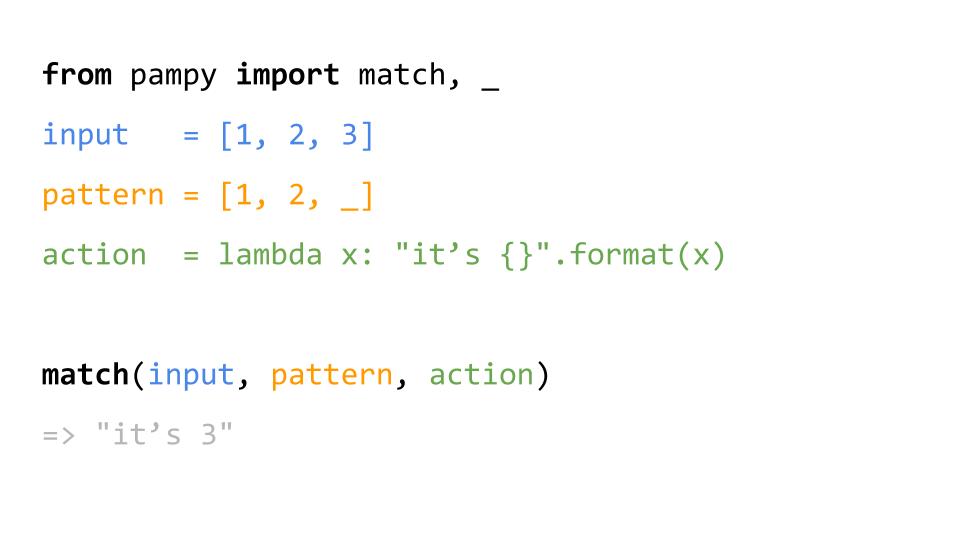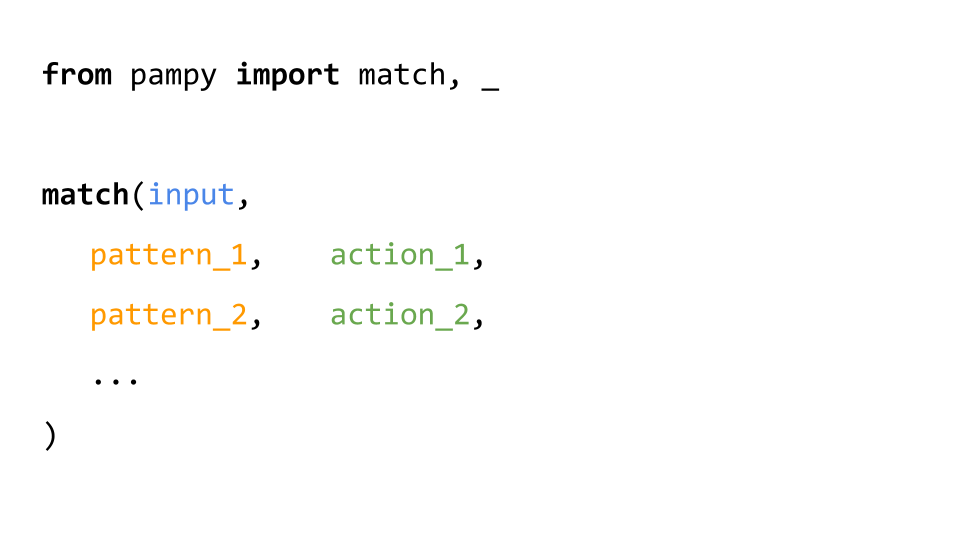189a34b Mar 10, 2019
4 contributors

### Users who have contributed to this file

326 lines (244 sloc) 12.5 KB# Pampy: Pattern Matching for Python

Pampy is pretty small (150 lines), reasonably fast, and often makes your code more readable and hence easier to reason about. There is also a JavaScript version, called Pampy.js.## You can write many patterns

Patterns are evaluated in the order they appear.## You can write Fibonacci

The operator _ means "any other case I didn't think of".

```from pampy import match, _

def fibonacci(n):
return match(n,
1, 1,
2, 1,
_, lambda x: fibonacci(x-1) + fibonacci(x-2)
)```

## You can write a Lisp calculator in 5 lines

```from pampy import match, REST, _

def lisp(exp):
return match(exp,
int,                lambda x: x,
callable,           lambda x: x,
(callable, REST),   lambda f, rest: f(*map(lisp, rest)),
tuple,              lambda t: list(map(lisp, t)),
)

plus = lambda a, b: a + b
minus = lambda a, b: a - b
from functools import reduce

lisp((plus, 1, 2))                 	# => 3
lisp((plus, 1, (minus, 4, 2)))     	# => 3
lisp((reduce, plus, (range, 10)))       # => 45```

## You can match so many things!

```match(x,
3,              "this matches the number 3",

int,            "matches any integer",

(str, int),     lambda a, b: "a tuple (a, b) you can use in a function",

[1, 2, _],      "any list of 3 elements that begins with [1, 2]",

{'x': _},       "any dict with a key 'x' and any value associated",

_,              "anything else"
)```

## You can match [HEAD, TAIL]

```from pampy import match, HEAD, TAIL, _

x = [1, 2, 3]

match(x, [1, TAIL],     lambda t: t)            # => [2, 3]

match(x, [HEAD, TAIL],  lambda h, t: (h, t))    # => (1, [2, 3])
```

`TAIL` and `REST` actually mean the same thing.

## You can nest lists and tuples

```from pampy import match, _

x = [1, [2, 3], 4]

match(x, [1, [_, 3], _], lambda a, b: [1, [a, 3], b])           # => [1, [2, 3], 4]```

## You can nest dicts. And you can use _ as key!

```pet = { 'type': 'dog', 'details': { 'age': 3 } }

match(pet, { 'details': { 'age': _ } }, lambda age: age)        # => 3

match(pet, { _ : { 'age': _ } },        lambda a, b: (a, b))    # => ('details', 3)```

It feels like putting multiple _ inside dicts shouldn't work. Isn't ordering in dicts not guaranteed ? But it does because in Python 3.7, dict maintains insertion key order by default

## You can match class hierarchies

```class Pet:          pass
class Dog(Pet):     pass
class Cat(Pet):     pass
class Hamster(Pet): pass

def what_is(x):
return match(x,
Dog, 		'dog',
Cat, 		'cat',
Pet, 		'any other pet',
_, 		'this is not a pet at all',
)

what_is(Cat())      # => 'cat'
what_is(Dog())      # => 'dog'
what_is(Hamster())  # => 'any other pet'
what_is(Pet())      # => 'any other pet'
what_is(42)         # => 'this is not a pet at all'```

## Using Dataclasses

Pampy supports Python 3.7 dataclasses. You can pass the operator `_` as arguments and it will match those fields.

```@dataclass
class Pet:
name: str
age: int

pet = Pet('rover', 7)

match(pet, Pet('rover', _), lambda age: age)                    # => 7
match(pet, Pet(_, 7), lambda name: name)                        # => 'rover'
match(pet, Pet(_, _), lambda name, age: (name, age))            # => ('rover', 7)```

## Using typing

Pampy supports typing annotations.

```class Pet:          pass
class Dog(Pet):     pass
class Cat(Pet):     pass
class Hamster(Pet): pass

timestamp = NewType("year", Union[int, float])

def annotated(a: Tuple[int, float], b: str, c: E) -> timestamp:
pass

match((1, 2), Tuple[int, int], lambda a, b: (a, b))             # => (1, 2)
match(1, Union[str, int], lambda x: x)                          # => 1
match('a', Union[str, int], lambda x: x)                        # => 'a'
match('a', Optional[str], lambda x: x)                          # => 'a'
match(None, Optional[str], lambda x: x)                         # => None
match(Pet, Type[Pet], lambda x: x)                              # => Pet
match(Cat, Type[Pet], lambda x: x)                              # => Cat
match(Dog, Any, lambda x: x)                                    # => Dog
match(Dog, Type[Any], lambda x: x)                              # => Dog
match(15, timestamp, lambda x: x)                               # => 15
match(10.0, timestamp, lambda x: x)                             # => 10.0
match([1, 2, 3], List[int], lambda x: x)                        # => [1, 2, 3]
match({'a': 1, 'b': 2}, Dict[str, int], lambda x: x)            # => {'a': 1, 'b': 2}
match(annotated,
Callable[[Tuple[int, float], str, Pet], timestamp], lambda x: x
)                                                               # => annotated```

For iterable generics actual type of value is guessed based on the first element.

```match([1, 2, 3], List[int], lambda x: x)                        # => [1, 2, 3]
match([1, "b", "a"], List[int], lambda x: x)                    # => [1, "b", "a"]
match(["a", "b", "c"], List[int], lambda x: x)                  # raises MatchError
match(["a", "b", "c"], List[Union[str, int]], lambda x: x)      # ["a", "b", "c"]

match({"a": 1, "b": 2}, Dict[str, int], lambda x: x)            # {"a": 1, "b": 2}
match({"a": 1, "b": "dog"}, Dict[str, int], lambda x: x)        # {"a": 1, "b": "dog"}
match({"a": 1, 1: 2}, Dict[str, int], lambda x: x)              # {"a": 1, 1: 2}
match({2: 1, 1: 2}, Dict[str, int], lambda x: x)                # raises MatchError
match({2: 1, 1: 2}, Dict[Union[str, int], int], lambda x: x)    # {2: 1, 1: 2}```

Iterable generics also match with any of their subtypes.

```match([1, 2, 3], Iterable[int], lambda x: x)                     # => [1, 2, 3]
match({1, 2, 3}, Iterable[int], lambda x: x)                     # => {1, 2, 3}
match(range(10), Iterable[int], lambda x: x)                     # => range(10)

match([1, 2, 3], List[int], lambda x: x)                         # => [1, 2, 3]
match({1, 2, 3}, List[int], lambda x: x)                         # => raises MatchError
match(range(10), List[int], lambda x: x)                         # => raises MatchError

match([1, 2, 3], Set[int], lambda x: x)                          # => raises MatchError
match({1, 2, 3}, Set[int], lambda x: x)                          # => {1, 2, 3}
match(range(10), Set[int], lambda x: x)                          # => raises MatchError```

For Callable any arg without annotation treated as Any.

```def annotated(a: int, b: int) -> float:
pass

def not_annotated(a, b):
pass

def partially_annotated(a, b: float):
pass

match(annotated, Callable[[int, int], float], lambda x: x)     # => annotated
match(not_annotated, Callable[[int, int], float], lambda x: x) # => raises MatchError
match(not_annotated, Callable[[Any, Any], Any], lambda x: x)   # => not_annotated
match(annotated, Callable[[Any, Any], Any], lambda x: x)       # => raises MatchError
match(partially_annotated,
Callable[[Any, float], Any], lambda x: x
)                                                              # => partially_annotated```

TypeVar is not supported.

## All the things you can match

As Pattern you can use any Python type, any class, or any Python value.

The operator `_` and built-in types like `int` or `str`, extract variables that are passed to functions.

Types and Classes are matched via `instanceof(value, pattern)`.

`Iterable` Patterns match recursively through all their elements. The same goes for dictionaries.

Pattern Example What it means Matched Example Arguments Passed to function NOT Matched Example
`"hello"` only the string `"hello"` matches `"hello"` nothing any other value
`None` only `None` `None` nothing any other value
`int` Any integer `42` `42` any other value
`float` Any float number `2.35` `2.35` any other value
`str` Any string `"hello"` `"hello"` any other value
`tuple` Any tuple `(1, 2)` `(1, 2)` any other value
`list` Any list `[1, 2]` `[1, 2]` any other value
`MyClass` Any instance of MyClass. And any object that extends MyClass. `MyClass()` that instance any other object
`_` Any object (even None) that value
`ANY` The same as `_` that value
`(int, int)` A tuple made of any two integers `(1, 2)` `1` and `2` (True, False)
`[1, 2, _]` A list that starts with 1, 2 and ends with any value `[1, 2, 3]` `3` `[1, 2, 3, 4]`
`[1, 2, TAIL]` A list that start with 1, 2 and ends with any sequence `[1, 2, 3, 4]` `[3, 4]` `[1, 7, 7, 7]`
`{'type':'dog', age: _ }` Any dict with `type: "dog"` and with an age `{"type":"dog", "age": 3}` `3` `{"type":"cat", "age":2}`
`{'type':'dog', age: int }` Any dict with `type: "dog"` and with an `int` age `{"type":"dog", "age": 3}` `3` `{"type":"dog", "age":2.3}`
`re.compile('(\w+)-(\w+)-cat\$')` Any string that matches that regular expression expr `"my-fuffy-cat"` `"my"` and `"puffy"` `"fuffy-dog"`
`Pet(name=_, age=7)` Any Pet dataclass with `age == 7` `Pet('rover', 7)` `['rover']` `Pet('rover', 8)`
`Any` The same as `_` that value
`Union[int, float, None]` Any integer or float number or None `2.35` `2.35` any other value
`Optional[int]` The same as `Union[int, None]` `2` `2` any other value
`Type[MyClass]` Any subclass of MyClass. And any class that extends MyClass. `MyClass` that class any other object
`Callable[[int], float]` Any callable with exactly that signature `def a(q:int) -> float: ...` that function `def a(q) -> float: ...`
`Tuple[MyClass, int, float]` The same as `(MyClass, int, float)`
`Mapping[str, int]` Any subtype of `Mapping` acceptable too any mapping or subtype of mapping with string keys and integer values `{'a': 2, 'b': 3}` that dict `{'a': 'b', 'b': 'c'}`
`Iterable[int]` Any subtype of `Iterable` acceptable too any iterable or subtype of iterable with integer values `range(10)` and `[1, 2, 3]` that iterable `['a', 'b', 'v']`

## Using default

By default `match()` is strict. If no pattern matches, it raises a `MatchError`.

You can instead provide a fallback value using `default` to be used when nothing matches.

``````>>> match([1, 2], [1, 2, 3], "whatever")
MatchError: '_' not provided. This case is not handled: [1, 2]

>>> match([1, 2], [1, 2, 3], "whatever", default=False)
False
``````

## Using Regular Expressions

Pampy supports Python's Regex. You can pass a compiled regex as pattern, and Pampy is going to run `patter.search()`, and then pass to the action function the result of `.groups()`.

```def what_is(pet):
return match(pet,
re.compile('(\w+)-(\w+)-cat\$'),     lambda name, my: 'cat '+name,
re.compile('(\w+)-(\w+)-dog\$'),     lambda name, my: 'dog '+name,
_,                                  "something else"
)

what_is('fuffy-my-dog')     # => 'dog fuffy'
what_is('puffy-her-dog')    # => 'dog puffy'
what_is('carla-your-cat')   # => 'cat carla'
what_is('roger-my-hamster') # => 'something else'```

## Install for Python3

Pampy works in Python >= 3.6 Because dict matching can work only in the latest Pythons.

To install it:

`\$ pip install pampy`

or `\$ pip3 install pampy`

## If you really must use Python2

Pampy is Python3-first, but you can use most of its features in Python2 via this backport by Manuel Barkhau:

`pip install backports.pampy`

`from backports.pampy import match, HEAD, TAIL, _`
You can’t perform that action at this time.